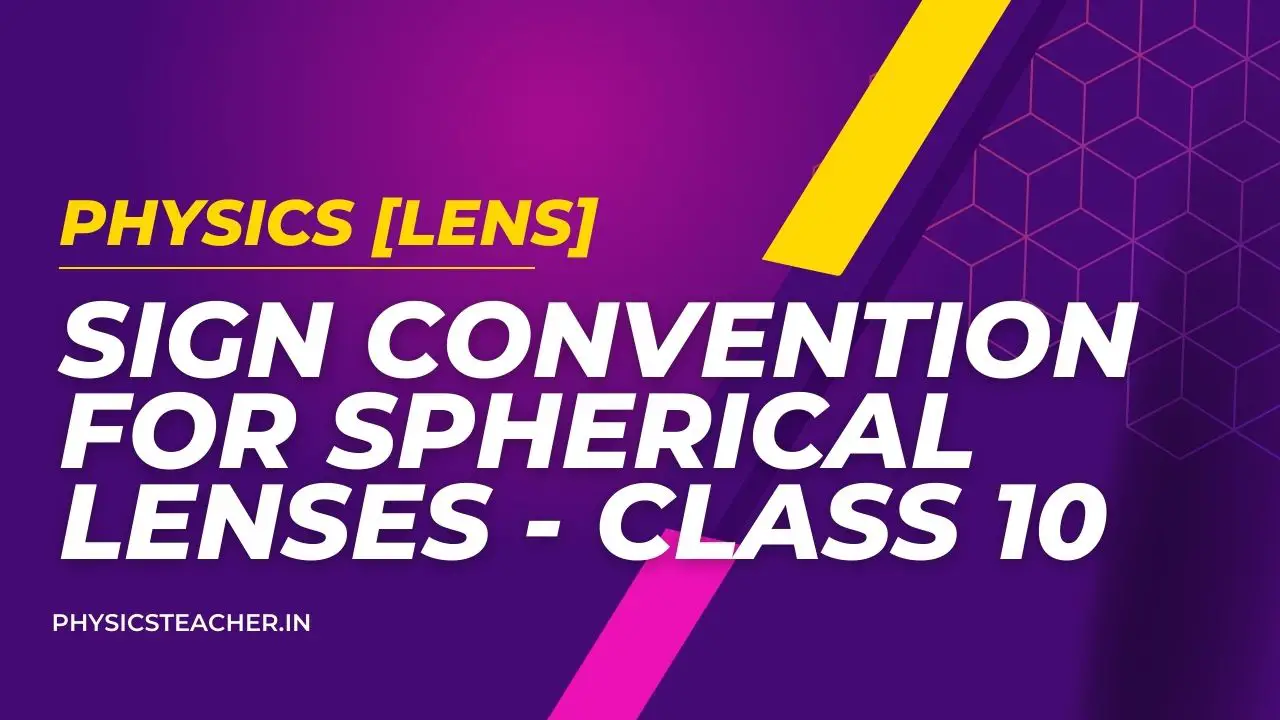# Sign convention for Spherical Lenses – class 10

Last updated on July 14th, 2023 at 11:26 am

Here we will present the New Cartesian Sign Convention for spherical lenses for measuring various distances in the ray diagrams of spherical lenses (convex lenses and concave lenses).

Also read side by side: Sign convention for Spherical Mirrors

## Sign convention for spherical lenses

According to the New Cartesian Sign, the sign Convention for spherical lenses goes like the following:
(i) All the distances are measured from the optical centre of the lens.
(ii) The distances measured (from the optical centre) in the same direction as that of incident light are taken as positive.
(iii) The distances measured (from the optical centre) against the direction of incident light are taken as negative.
(iv) The heights measured upward and perpendicular to the principal axis are taken as positive.
(v) The heights measured downward and perpendicular to the principal axis are taken as negative.

Sign convention for spherical lenses – explanation with diagram

The New Cartesian Sign Convention for lenses is shown in Figure 1.

The object is placed on the left side of the lens (as shown in Figure 1) so that the direction of incident light is from left to right.

Distances – sign convention of lenses

• All the distances measured from the optical centre of the lens to the right side will be considered positive (because they will be in the same direction as the incident light).
• On the other hand, all the distances measured from the optical centre of the lens to the left side are considered negative (because they are measured against the direction of incident light).

focal length – sign convention of lenses

On the basis of New Cartesian Sign Convention, the focal length of a convex lens is considered positive (and written with a plus sign).

On the other hand, the focal length of a concave lens is considered negative (and written with a minus sign).

The focal length of a convex lens is positive and that of a concave lens is negative.

Consequences of the new Cartesian sign convention for lenses

1) The focal length of a convex lens is positive and that of a concave lens is negative.

2) Object distance u is always negative.

3) The distance of real image is positive and that of virtual image is negative. (for lenses)

4) The object height h is always positive. Height h’ of virtual erect image is positive and that of real inverted image is negative.

5) The linear magnification, m = h’/h is positive for a virtual image and negative for a real image.

Please note that the sign convention for spherical lenses is very similar to the sign convention for spherical mirrors.